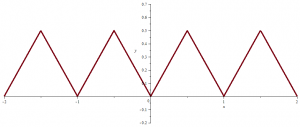A function that is everywhere continuous and nowhere differentiable

Let $$f_1(x) = |x|$$ for $$| x | \le \frac{1}{2}$$, and let $$f_1$$ be defined for other values of $$x$$ by periodic continuation with period $$1$$. $$f_1$$ graph looks like following picture:$$f_1$$ is continuous everywhere and differentiable on $$\mathbb{R} \setminus \mathbb{Z}$$.

For $$n > 1$$ define $$f_n(x)=\frac{f_1(4^{n-1} x)}{4^{n-1}}$$, so that for every positive integer $$n$$, $$f_n$$ is a continuous periodic function of period $$\frac{1}{4^{n-1}}$$ and maximum value $$\frac{1}{2 \cdot 4^{n-1}}$$.

Finally, define $$f$$ for $$x \in \mathbb{R}$$ by:
$f(x) = \sum_{n=1}^{+ \infty} f_n(x) = \sum_{n=1}^{+ \infty} \frac{f_1(4^{n-1} x)}{4^{n-1}}$

The function series $$(f_n)_{n \ge 1}$$ is normally convergent and therefore $$f$$ is continuous.

$$f$$ is nowhere differentiable

To see this let $$a$$ be an arbitrary real number.
For any positive integer $$n$$, choose $$h_n$$ to be either $$\frac{1}{4^n}$$ or $$-\frac{1}{4^n}$$ so that $$|f_n(a+h_n)-f_n(a)|=|h_n|$$. This is possible because $$h_n$$ is equal to a quarter of the period of $$f_n$$ while $$f_n$$ is a linear function of slope $$\pm 1$$ in an interval of length $$2 h_n$$ containing $$a$$.
$$|f_m(a+h_n)-f_m(a)|$$ has this same value $$|h_n|$$ for all $$m \le n$$, and vanishes for $$m > n$$. Hence the difference quotient $$\frac{f(a+h_n)-f(a)}{h_n}$$ is an integer whose parity is the one of $$n$$. It follows that:
$\lim\limits_{n \to \infty} \frac{f(a+h_n)-f(a)}{h_n}$ cannot exist, which proves that $$f$$ is not differentiable at point $$a$$ which was arbitrarily chosen.

$$f$$ is also nowhere monotonic

For any point of the form $$a=\frac{k}{4^m}$$, where $$k$$ is an integer and $$m$$ a positive integer, we have $$f_n(a)=0$$ for $$n > m$$ and hence
$f(a)=\sum_{i=1}^m f_i(a)$ Let $$h_m=\frac{1}{4^{2m+1}}$$, $$h_m$$ is a period of $$f_n$$ for $$n > 2m+1$$ and therefore $$f_n(a+h_m)=0$$. Hence \begin{aligned} f(a+h_m)-f(a) &= \sum_{n=1}^m (f_n(a+h_m)-f_n(a)) + \sum_{n=m+1}^{2m+1} f_n(a+h_m)\\ &\ge -m h_m + (m+1)h_m >0 \end{aligned} Similarly $$f(a-h_m)-f(a) \ge h_m > 0$$. As numbers of the form $$\frac{k}{4^m}$$ are dense, $$f$$ cannot be monotonic in no open interval.

The first example of a continuous real function nowhere differentiable was shown by Karl Weierstrass in 1872. See Wikipedia – Weierstrass function for more details.